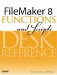# StDev()

Category: Aggregate

 Syntax: StDev ( field {; field...} )

Parameters:

fieldAny related field, repeating field, or set of non-repeating fields that represent a collection of numbers. Parameters in curly braces { } are optional.

Data type returned: Number

Description:

Returns the standard deviation of the non-blank values represented in the parameter list. Standard deviation is a statistical measurement of how spread out a collection of values is. In a normal distribution, about 68% of the values are within one standard deviation of the mean, and about 95% are within two standard deviations of the mean.

The difference between the StDevP() and StDev() functions is that StDev divides the sum of the squares by n-1 instead of by n.

StDev() can also be calculated as the square root of the Variance() of a set of numbers.

Examples:

There are several ways you can manually calculate the standard deviation of a set of numbers. One is to take the square root of the sum of the squares of each values distance from the mean, divided by n-1, where n is the number of values in the set.

For instance, given the set of numbers 8, 10, and 12, the mean of this set is 10. The distances of each value from the mean are therefore -2, 0, and 2. The squares of these are 4, 0, and 4. The sum of the squares is 8. The standard deviation of the population is Sqrt (8/(3-1)), which is 2.

``` StDev (8; 10; 12)
```

returns 2.

Given a portal that contains a field called Scores with the following values (64, 72, 75, 59, 67),

``` StDev (People::Scores)
```

returns 6.35.FileMaker 8 Functions and Scripts Desk Reference
ISBN: 0789735113
EAN: 2147483647
Year: 2004
Pages: 352